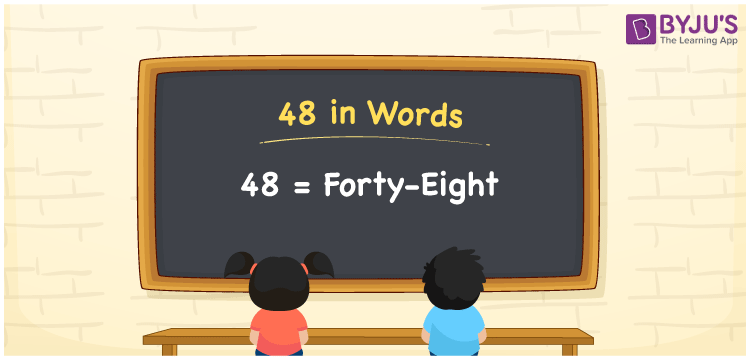# 48 in Words

We can express 48 in words as Forty-Eight. The number 48 is used to express a count that is equal to that value. For example, if you have 48 rupees in your wallet. You can write it as “I have Forty-Eight rupees in my wallet”. 48 is a cardinal number or counting number. First, we need to check with the place values of individual digits to express the number 48 in words. Let us learn the spelling of 48 in English here.

 48 in words Forty-Eight Forty-Eight in numerical form 48

## 48 in English Words

All the numbers in words are generally written using the English alphabet. Therefore, we can write the number 48 in English as “Forty-Eight”.## How to Write 48 in Words?

We know that the place value represents the position of a digit in a number. Hence, we can write 48 in words using the place value method.

 Tens Ones 4 8

Thus, we can write the expanded form as:

4 x Tens + 8 x Ones

= 4 x 10 + 8 x 1

= 40 + 8

= 48

= Forty-Eight

Therefore, 48 in words is written as Forty-Eight.

Interesting way of writing 48 in words

4 = Four

8 = Eight

40 = Forty

40 + 8 = 48

Forty + Eight = Forty-Eight

Hence, 48 in words = Forty-Eight

48 is a natural number whose successor is 49 and the predecessor is 47

• 48 in words – Forty-Eight
• Is 48 an odd number? – No
• Is 48 an even number? – Yes
• Is 48 a perfect square number? – No
• Is 48 a perfect cube number? – No
• Is 48 a prime number? – No
• Is 48 a composite number? – Yes

## Frequently Asked Questions on 48 in Words

Q1

### How do you write 48 in words?

48 in words is written as Forty-Eight.
Q2

### What is the value of 48 minus 8?

48 minus 8 = 48 – 8 = 40. Hence, the value of 48 minus 8 is equal to 40.
Q3

### 48 is a prime number. True or False.

False. 48 has more than two factors. Hence, it is a composite number.